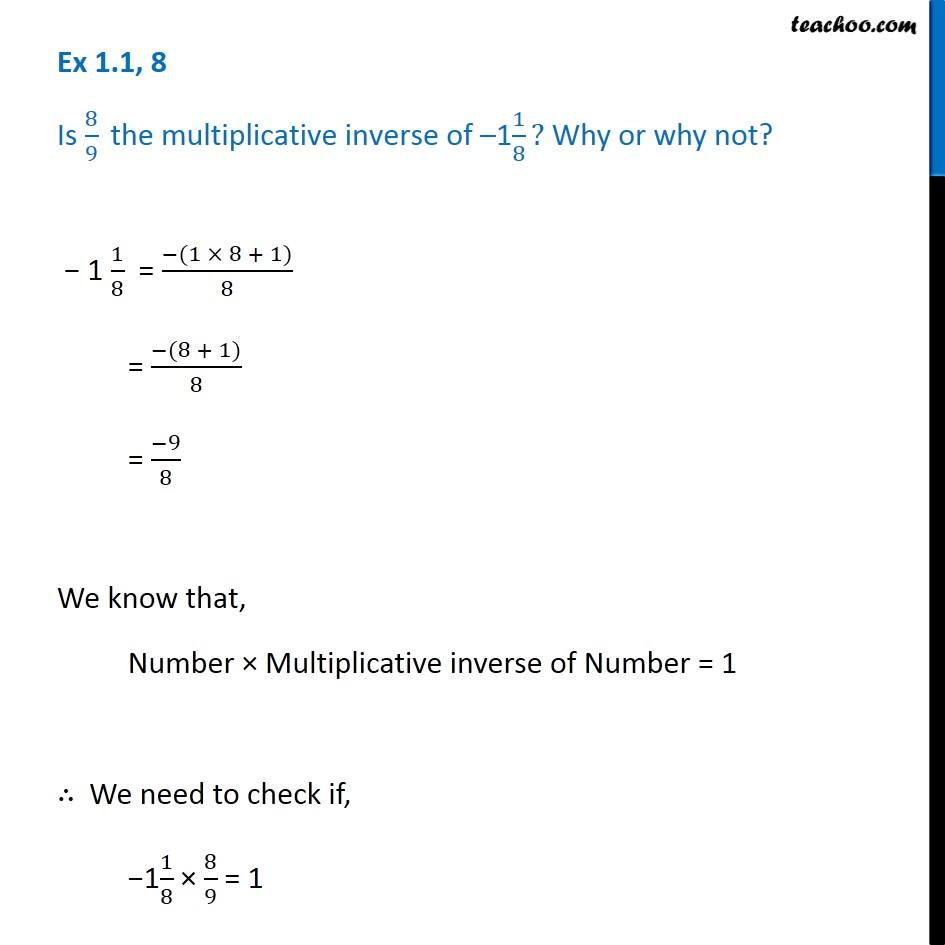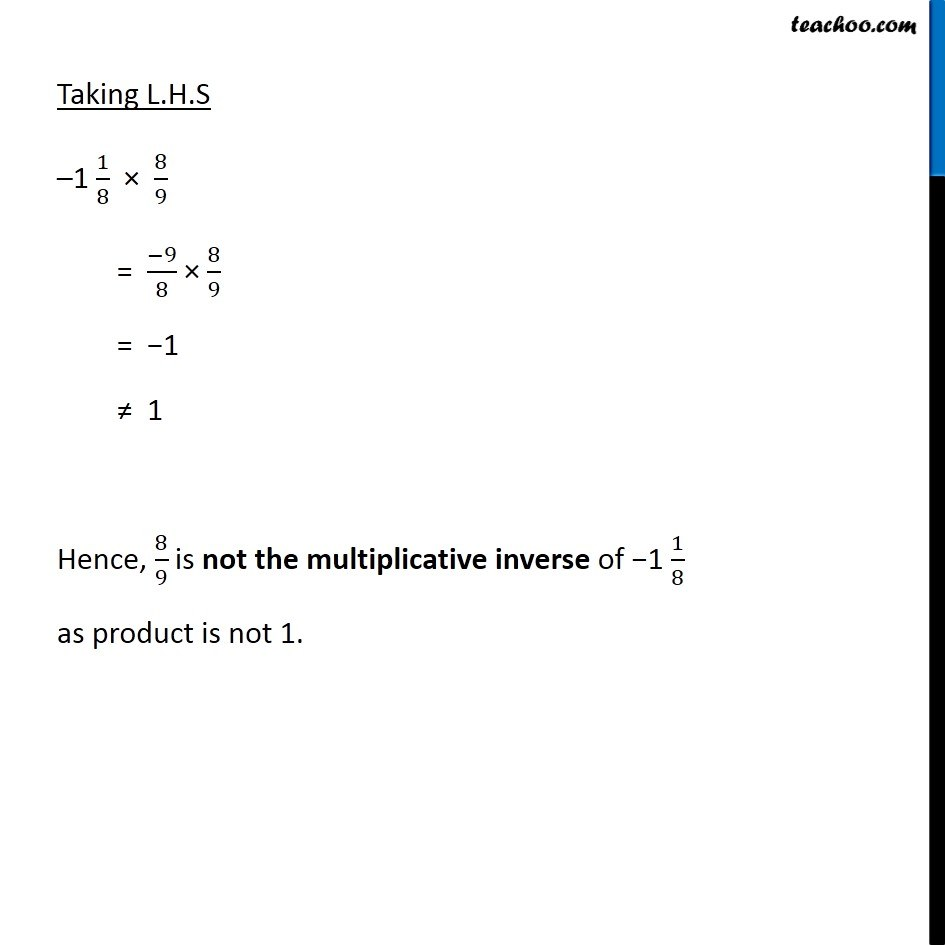Ex 1.1

Chapter 1 Class 8 Rational Numbers
Serial order wiseGet live Maths 1-on-1 Classs - Class 6 to 12

### Transcript

Ex 1.1, 8 Is 8/9 " the multiplicative inverse of" –11/8? Why or why not? − 1 1/8 = (−(1 × 8 + 1))/8 = (−(8 + 1))/8 = (−9)/8 We know that, Number × Multiplicative inverse of Number = 1 ∴ We need to check if, −11/8 × 8/9 = 1 Taking L.H.S –1 1/8 × 8/9 = (−9)/8 × 8/9 = −1 ≠ 1 Hence, 8/9 is not the multiplicative inverse of −1 1/8 as product is not 1.